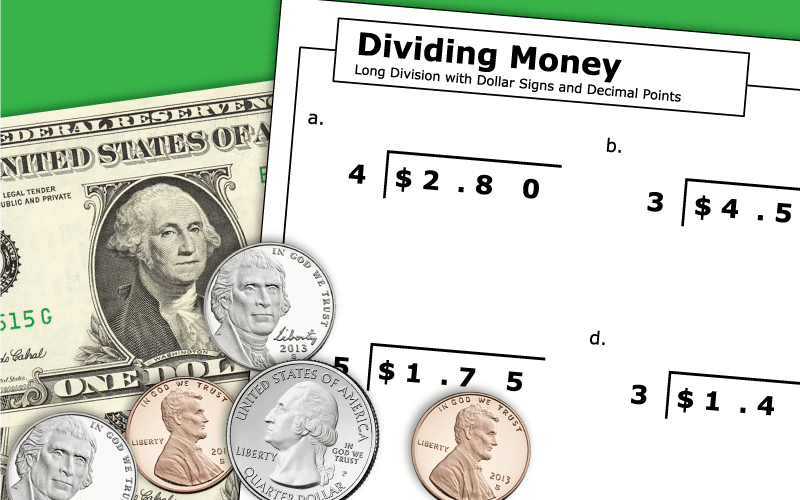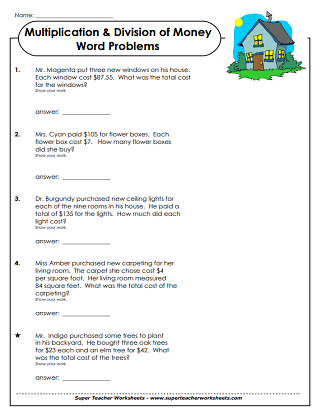# Long Division - Dividing Money

Below you'll find a series of worksheets, task cards, and activities for teaching long division with money.Long division of money amounts; No remainders; Popsicle picture and word problem.
For each problem, decide whether to multiply or divide, then solve.  Includes money problems with 3 and 4-digit numbers.
With this worksheet students can practice dividing money amounts by 1-digit divisors.
Use this variety worksheet to help students practice dividing money amounts.
Use this variety worksheet to help students practice dividing money amounts in the hundreds and thousands by 1- and 2-digit divisors.
Find the quotient for each of these 30 division problems involving money. Use these cards as a math learning center, morning challenge, or scavenger hunt.

## Long DivisionWorksheet Generator

Division Worksheet Generator

Create your own long division worksheets! You choose the number of digits in the dividend and the divisor. Also tell if you would like remainders.

More Long Division Worksheets

STW has a massive collection of long division printables. Choose from 2, 3, or 4-digit dividends.

## Sample Worksheet ImagesMy Account
Site Information